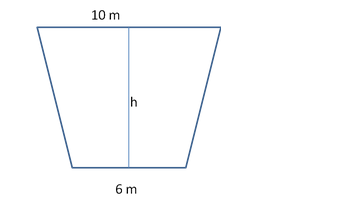# The shape of the cross section of a canal is a trapezium.`
Question:

The shape of the cross section of a canal is a trapezium. If the canal is 10 m wide at the top, 6 m wide at the bottom and the area of its cross section is 640 m2, find the depth of the canal.

Solution:Area of the canal $=640 \mathrm{~m}^{2}$

Area of trapezium $=\frac{1}{2} \times($ Sum of parallel sides $) \times($ Distance between them $)$

$\Rightarrow 640=\frac{1}{2} \times(10+6) \times h$

$\Rightarrow \frac{1280}{16}=h$

$\Rightarrow h=80 \mathrm{~m}$

Therefore, the depth of the canal is 80 m.